## eScience Lectures Notes : Mathematics for Computer Graphics

Slide 1 : 1 / 23 : Mathematics for Computer Graphics

# Mathematics for Computer Graphics

## Ref : Computer Graphics / Foley - VanDam - Feiner - Hughes

This part is somewhat theoritical, but it should not be too difficult with a simple mathematical background

Slide 2 : 2 / 23 : Why Mathematics ?

# Why Mathematics ?

• ### For more reasons, See Understanding Mathematics

Slide 3 : 3 / 23 : Vector Space

# Vector Space

## Set of elements called vectors, with 2 operations with certain properties:

Usually we will note a vector in boldfaced letters or with an arrow above it

### 1 ) Addition of vectors : commutative, associative, with an identity element and each element must have inverses

• u + v = v + u
• u + ( v + w ) = (u + v ) + w
• there is 0 such as for any vector v, 0 + v = v
• for every vector v, there is another vector w such as v + w = 0 . w is written " -v "

### 2 ) Scalar Multiplication : associative and distributive

• (ab)v = a (bv)
• 1v = v
• (a + b)v = av + bv
• a(v + w) =av + aw

### Given those two operations, we may define a "linear combination" of a set of vector v1, ... vn : any vector of the form

a1v1 + a2v2 + ... anvn

### Sub example : R3 :

```/ r1                     / 1   / 4   / 5
| r2      ......   ex :  | 3 + | 5 = |12
\ r3                     \ 6   \ 2   \ 8```

Slide 4 : 4 / 23 : Plane Vector Splace

# One particular Vector Space : The Plane

### The vector 0 : the one which is matched with the chosen origin

Slide 5 : 5 / 23 : Affine Space

# Affine Space

## A set in which geometric operations make sense, but in which there is no distinguished point

P - Q = v

P = Q + v

### Properties to satisfy

(P + v) + u = P + (v + u)

P + u = P if and only if u = 0

## Affine combination of the points P and Q by the real number t : a point such as :

P + t (Q-P) ....... if a + b = 1 ... new notation : aP + bQ <=> P + b (Q-P)

### NB : convex combination <=> 0 <= t <= 1

if t1 + t2 + ... tn = 1

t1 P1 + t2 P2 + ... tn Pn    <=>    P1 + t2 (P2 - P1) + ... tn (Pn - P1)

NB : convex affine combination <=> 0 <= ti <= 1

Slide 6 : 6 / 23 : Various other curiosities

# Various other curiosities

## Vector and affine linear subspace are resp. vector and affine space

V : Non empty subset which is stable through the addition and the multiplication by a real

A : such as the set of the difference between any element of A is a linear vector subspace

```
/ x
| y
| z
\ 0
```

## Affine Subspace of R4 : standard affine 3 space

```
/ x                        / x1 - x2
| y              v1 - v2 = | y1 - y2   belongs to the previous
| z                        | z1 - z2   vector subspace of R4
\ 1                        \ 0
```

Slide 7 : 7 / 23 : Cartesian coordinate system

# Cartesian coordinate system 2D

## Two usual cartesian reference frames

### "Maths" frame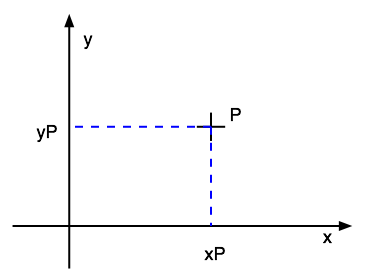### "Computer Graphics" frame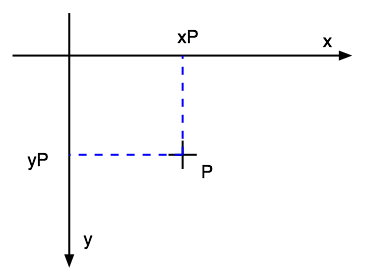### PS. : "Cartesian" comes from René Descartes

Slide 8 : 8 / 23 : Cartesian coordinate system 3D

# Cartesian coordinate system 3D

## 3 fingers or thumb or corkscrew rules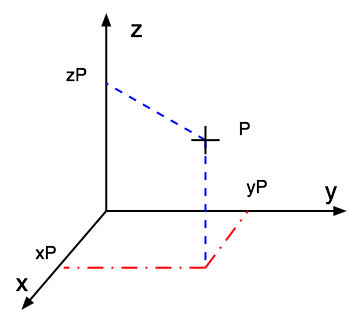###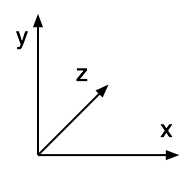Left handed system

```   / a1     / a2               / a2-a1
M | b1   P | b2      u = MP = | b2-b1
\ c1     \ c2               \ c2-c1
```

Slide 9 : 9 / 23 : Dot Product and Distance

# Dot Product and Distance (2D, 3D, ...)

### a.k.a. Scalar Product

```        / x1   / x2
u.v =  | y1 . | y2  =  x1.x2 + y1.y2 + z1.z2
\ z1   \ z2
```

## Distance between M and P :

u = MP

### v = 0  <=>  ||v|| = 0  <=>  v.v = 0

```   / a1     / a2               / a2-a1
M | b1   P | b2      u = MP = | b2-b1
\ c1     \ c2               \ c2-c1
```

Slide 10 : 10 / 23 : Dot Product and Angle

# Dot Product and Angle

## (or u = 0, or v = 0 ...)

### Length = u.v / || u || = (u/||u||).v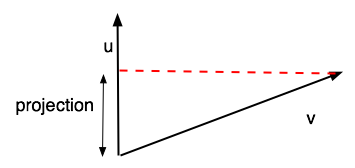Slide 11 : 11 / 23 : Cross Product

# Cross Product

### a.k.a. the vector product

```                  / x1   / x2     / y1.z2 - y2.z1
u x v = u ^ v =  | y1 x | y2  =  | x2.z1 - x1.z2
\ z1   \ z2     \ x1.y2 - x2.y1```

### u x v is a vector perpendicular to both u and v , which direction is given by the usual right handed rule, and the length, by :

|| u x v || = || u || . || v || . sin (uˆv)

### now we are able to get the angle ...

Before going further, let's check those properties (again ?)

#### if ( i, j, k ) are the set of vectors that define your right handed three-dimensional coordinate system, then k = i x j

```                  / 1   / 0     / 0.0 - 1.0     / 0
i x j = i ^ j =  | 0 x | 1  =  | 0.0 - 1.0  =  | 0  =  k
\ 0   \ 0     \ 1.1 - 0.0     \ 1```

### Other simple problem : How to compute the normal to a plane ?

Slide 12 : 12 / 23 : How to get an orthogonal Vector .... in 2D

# How to get an orthogonal Vector .... in 2D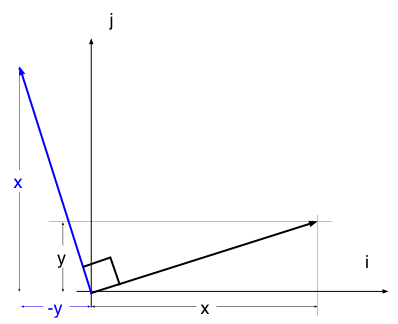```       | x              | -y            |  y
v = | y    =>    w = |  x    or -w = | -x```

Slide 13 : 13 / 23 : Orthogonal Vector .... in 3D

# How to get an new cartesian coordinate system in 3D, starting from one vector.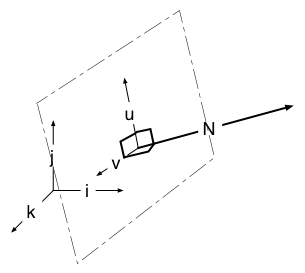## Let's just define, arbitrarily,        u = k x N / || k x N ||

### With u and v, we've got an orthogonal basis (hamel basis) within the plan normal to N

#### What if N and j are orthogonal ?

then N and j are not parallel => N x j not null => u = N x j

What about continuity issues (would it be better to use i : N x i ?)

An application : the envelope of a path

Slide 14 : 14 / 23 : Locus

# Where could I Be ?

## Locus of point defined by a constant view angle from 2 fixed points.

#### Set of point defined by the fact that they form a given angle with two fixed points (A et B, of the Euclidian Plane).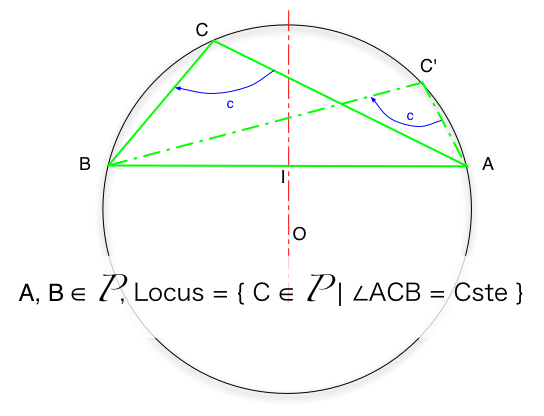NB : Be careful to be coherent with the orientation of your angles, and that the construction is not the same if the angle is acute (<90°) or obtuse (>90°)

Slide 15 : 15 / 23 : Locus

# Locus of point defined by a constant view angle from 2 fixed points

### Set of point defined by the fact that they form a given angle with two fixed points (A et B, of the Euclidian Plane).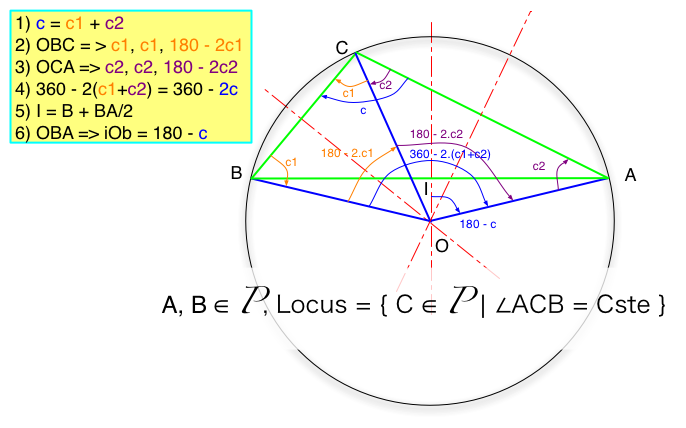0) Given A, B and an angle c defined between 0 and 180°, positive from A to B. We will demonstrate that the set of point C such as aCb = c is indeed the arc AB (which arc AB is defined by the relative value of the angle compared to 90°). To achieve that, we consider one given C point that verify aCb = c and we look at the centre of the circum(scribed )circle for the triangle (ABC). We will indeed show that this circle can be describe without referring to C, but just AB and the angle c, and that therefore the possible set of C points are all on that circle.

1) Given ABC, it is well known that the circumscribed circle has its circumcenter O at the intersection of the 3 perpendicular bisectors of the triangle. Let's consider the two triangles (OCB) and (OAC) which share the common edge [OC]. c = c1 + c2

2) In all triangles, the somme of the angles equal to180. As O is on the perpendicular bisector of [CB], then (OCB) is a isosceles and therefore oCb = cBo = c1. From those two fact the last angle bOc = 180 - 2.c1

3) Likewise, in the isosceles triangle (OAC), we get cOa = 180 - 2.c2

4) bOa = bOc + cOa = 180 - 2.c1 + 180 - 2.c2 = 360 - 2 c

5) I is the middle of [AB], and then on its same perpendicular bisector as O. Therefore (IOB) is rectangle in I. Knowing I, A and the angle iOa = bOa / 2 = 180 - c, it is possible to defined O, without any reference to C. We have indeed demonstrate that given two fixed point A and B and an oriented angle c, the set of point C such as aCb = c is on the circle defined by its centre O and its radius OA.

NB. : Just note the effect of the orientation of the angle and its value relative to 90° to the selection of the part of the circle and the position of O relative to [AB]

PS. Question : why is the intersection of the 3 orthogonal bisectors the centre of the circumcircle ... ?

Slide 16 : 16 / 23 : Triangulation

# Almost Triangulation : why you need at least two measures to guess where you are ...

####Why we should use the 4 landmarks solution#### Triangulation : A method of fixing an unknown point (for example in navigation) by making it a vertex of a triangle whose other vertices and angles are known (Collins Dictionary of Mathematics).

Slide 17 : 17 / 23 : Triangulation with a compass

# Triangulation with a compass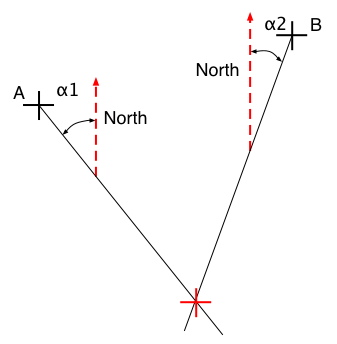### Then you need only 2 landmarks !

You indeed still use 3 landmarks, the last one only happen to be really, really far away (North direction) and this is just a special limit case of the previous situation (when the arc of the circle tend to a segment when N tend towards the North direction)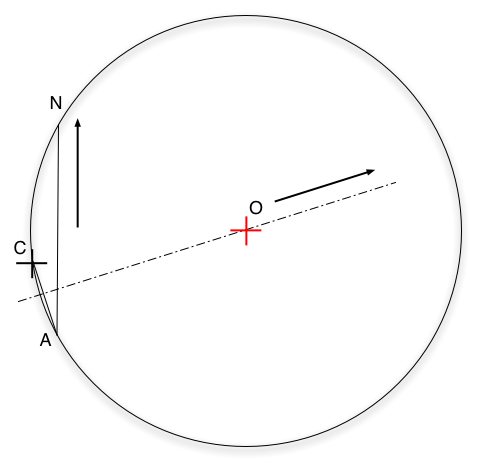Slide 18 : 18 / 23 : Intersection between two circles

# Intersection between two circles

### Given M(x,y,z) the intersection, then the rought way is ...

(1)     (x - x1)2 + (y - y1)2 = r12
(2)     (x - x2)2 + (y - y2)2 = r22
x2 - 2xx1 + x12 + y2 -2yy1 + y12 = r12
x2 - 2xx2 + x22 + y2 -2yy2 + y22 = r22
(1) - (2)      2x(x2 - x1) + 2y(y2 - y1) + x12 - x22  + y12 - y22 = r12 - r22
if x2 - x1 > Epsilon     x = ( r12 - r22 - ( 2y(y2 - y1) + x12 - x22  + y12 - y22) ) / 2(x2 - x1)     else y = ...

#### ...piece of cake but ...

Slide 19 : 19 / 23 : Intersection between two circles (2)

# Intersection between two circles (2)

### Given M(x,y,z) the intersection, then another way is ...O1O2 > r1 + r2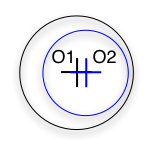O1O2 < (r1 - r2) and (r1>r2) NB.: what if r1 = r2 ?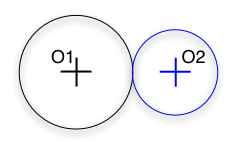O1O2 = r1 + r2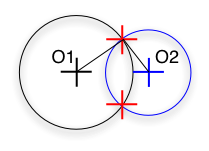r1 - r2  < O1O2 < r1 + r2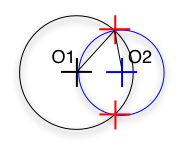r1 - r2  < O1O2 < r1 + r2r1 - r2  < O1O2 < r1 + r2

### NB. : when you see " = 0 " in Maths, you translate into "< Epsilon" in Computer

Slide 20 : 20 / 23 : Intersection between two circles (3)

# Intersection between two circles (3)

## M = O1 + O1 H +/- HM

Then O1H = x. O1O2/||O1O2||      then inverse -x and y of  O1O2/||O1O2|| vector to get an orthogonal vector

Slide 21 : 21 / 23 : Intersection between two circles (4)

# Intersection between two circles (4)

### AMB obtuse <=> MA . MB < 0

#### What if AMB is a right angle ?

Then we have to be sur of the sign of the angle

Slide 22 : 22 / 23 : Exercise from Last year Exam

# Exercise from Last year Exam

### Question 16 ( 8 marks ) :

• #### Considering that M is inside the triangle if and only if s >= 0, t >= 0 and s+t <= 1 draw your conclusion

Slide 23 : 23 / 23 : Exercise from Last year Exam